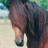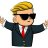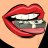# Multiple Time Frame (MTF) RSI Indicator for ThinkorSwim#### horserider

##### Well-known member
VIP
Chart time frame plus choice of 2 additional time frames RSI. Cloud between chart time frame RSI and next highest time frame RSI. User can input two higher time frames. Make sure higher time frames are higher than chart time frame.

Code:
``````#MTF RSI Three standard ToS RSI studies with a choice of time frame for second and third RSI. Cloud between chart and second RSI.
# RSI with agg periods by Horserider. 5/12/2019

declare lower;
#RSI
input length = 14;
input over_Bought = 80;
input over_Sold = 20;
input price = close;
input averageType = AverageType.WILDERS;

def NetChgAvg = MovingAverage(averageType, price - price, length);
def TotChgAvg = MovingAverage(averageType, AbsValue(price - price), length);
def ChgRatio = if TotChgAvg != 0 then NetChgAvg / TotChgAvg else 0;

plot RSI = 50 * (ChgRatio + 1);
plot OverSold = over_Sold;
plot OverBought = over_Bought;
plot lline = 50;

RSI.DefineColor("Positive and Up", Color.GREEN);
RSI.DefineColor("Positive and Down", Color.DARK_GREEN);
RSI.DefineColor("Negative and Down", Color.RED);
RSI.DefineColor("Negative and Up", Color.DARK_RED);
RSI.AssignValueColor(if RSI >= 50 then if RSI > RSI then RSI.Color("Positive and Up") else RSI.Color("Positive and Down") else if RSI < RSI then RSI.Color("Negative and Down") else RSI.Color("Negative and Up"));

OverSold.SetDefaultColor(GetColor(7));
OverBought.SetDefaultColor(GetColor(7));

# RSI 2
input length2 = 14;
input price2 = close;
input averageType2 = AverageType.WILDERS;
input agg = AggregationPeriod.DAY;

def c = close(period = agg);

def NetChgAvg2 = MovingAverage(averageType2, c - c, length2);
def TotChgAvg2 = MovingAverage(averageType2, AbsValue(c - c),length2);
def ChgRatio2 = if TotChgAvg2 != 0 then NetChgAvg2 / TotChgAvg2 else 0;

plot RSI2 = 50 * (ChgRatio2 + 1);

RSI2.DefineColor("Positive and Up", Color.GREEN);
RSI2.DefineColor("Positive and Down", Color.DARK_GREEN);
RSI2.DefineColor("Negative and Down", Color.RED);
RSI2.DefineColor("Negative and Up", Color.DARK_RED);
RSI2.AssignValueColor(if RSI2 >= 50 then if RSI2 > RSI2 then RSI2.Color("Positive and Up") else RSI2.Color("Positive and Down") else if RSI2 < RSI2 then RSI2.Color("Negative and Down") else RSI2.Color("Negative and Up"));
RSI2.setLineWeight(3);

# RSI 3
input length3 = 14;
input price3 = close;
input averageType3 = AverageType.WILDERS;
input agg3 = AggregationPeriod.WEEK;

def c3 = close(period = agg3);

def NetChgAvg3 = MovingAverage(averageType3, c3 - c3, length3);
def TotChgAvg3 = MovingAverage(averageType3, AbsValue(c3 - c3),length3);
def ChgRatio3 = if TotChgAvg3 != 0 then NetChgAvg3 / TotChgAvg3 else 0;

plot RSI3 = 50 * (ChgRatio3 + 1);

RSI3.DefineColor("Positive and Up", Color.GREEN);
RSI3.DefineColor("Positive and Down", Color.DARK_GREEN);
RSI3.DefineColor("Negative and Down", Color.RED);
RSI3.DefineColor("Negative and Up", Color.DARK_RED);
RSI3.AssignValueColor(if RSI3 >= 50 then if RSI3 > RSI3 then RSI3.Color("Positive and Up") else RSI3.Color("Positive and Down") else if RSI3 < RSI3 then RSI3.Color("Negative and Down") else RSI3.Color("Negative and Up"));
RSI3.setLineWeight(5);Last edited:
••SolidChiken, SmellyCat, ugaotrader and 5 others
S

#### SmellyCat

##### New member
Very helpful study you posted - Thanks for sharing!!
How have you found it most useful?#### horserider

##### Well-known member
VIP
Updated to make easier to read. Commented out the cloud and made line weights thicker for each higher time agg.

•#### markos

##### Well-known member
VIP
@horserider Somehow I missed this take on an indicator that's a staple of many traders' toolbox! Excellent, Thanks Markos#### horserider

##### Well-known member
VIP
@markos Wake UP !!! hahaha just kidding. Hope you can make some use of it.

R

#### roger80k

##### New member
Can anyone help me locate this type of indicator or custom code? Since the RSI indicator is more accurate on the longer time frame. Thanks

R

#### roger80k

##### New member
If I just want to add the mtf time frame script into existing RSI with divergence would the below code is sufficient or there is more to it?

input agg = AggregationPeriod.DAY;

Thanks#### horserider

##### Well-known member
VIP
Try it and see. Not sure what you want. Show the code if having trouble.

R

#### roger80k

##### New member
I just want to select a time frame of my choice and use it on 1-5 min chart without having multiple windows open

R

#### roger80k

##### New member
Ok i just tested the same RSI on 5 min chart vs and mtf code and its not identical. Can anyone help me with this please. This is the code below

Code:
``````plot Data = close;# RSI_With_Divergence

# Mobius

# V01.01.2013

#hint:<b>RSI with Divergence</b>

# Note: Install this as a new study. Save this study using the name above (the first line of code RSI_With_Divergence).

# To use this study as a scan; DO NOT TRY TO LOAD IT DIRECTLY IN THE SCANNER, IT WILL THROW AN ERROR MESSAGE. Go to the scan tab. Delete any existing scan criteria. Click Add Study Filter. Click the window under Criteria. In that drop down menu click Custom. Delete the existing study. Click Add Condition. Click the down arrow in the Select A Condition window. Click Study. Scroll down the List till you find RSI_With_Divergence and click it. Click on the Plot window and you can choose Dhigh or Dlow in addition to the default plot RSI. If you choose either of the divergence siganls choose is True from the center column. Click on the aggregation period at the top left and set the aggregation period you want scaned. Then click Save and when the popup window shows the warning that this is a custom scan chose OK. Now put the list of stocks you wish to scan in the Scan In box and chose any list you want that to intersect with. If you wish to make this a Dynamic WatchList, save this scan with a name such as RSI_With_Div_WL then in your Gadgets box click the little gear icon, locate the name of the scan you just saved and click it. As equities match the scan criteria they will populate the list.

declare lower;

input n = 14;        #hint nRSI: Periods or length for RSI

input Over_Bought = 70; #hint Over_Bought: Over Bought line

input Over_Sold = 30;   #hint Over_Sold: Over Sold line

def o = open;

def h = high;

def l = low;

def c = close;

def x = BarNumber();

def MidLine = 50;

def NetChgAvg = ExpAverage(c - c, n);

def TotChgAvg = ExpAverage(AbsValue(c - c), n);

def ChgRatio = if TotChgAvg != 0

then NetChgAvg / TotChgAvg

else 0;

plot RSI = 50 * (ChgRatio + 1);

RSI.AssignValueColor(if RSI < Over_Sold

then Color.YELLOW

else if RSI > Over_Bought

then Color.YELLOW

else CreateColor(25, 75, 250));

plot OverSold = Over_Sold;

plot OverBought = Over_Bought;

def bar = BarNumber();

def Currh = if RSI > OverBought

then fold i = 1 to Floor(n / 2)

with p = 1

while p

do RSI > GetValue(RSI, -i)

else 0;

def CurrPivotH = if (bar > n and

RSI == Highest(RSI, Floor(n / 2)) and

Currh)

then RSI

else Double.NaN;

def Currl = if RSI < OverSold

then fold j = 1 to Floor(n / 2)

with q = 1

while q

do RSI < GetValue(RSI, -j)

else 0;

def CurrPivotL = if (bar > n and

RSI == Lowest(RSI, Floor(n / 2)) and

Currl)

then RSI

else Double.NaN;

def CurrPHBar = if !IsNaN(CurrPivotH)

then bar

else CurrPHBar;

def CurrPLBar = if !IsNaN(CurrPivotL)

then bar

else CurrPLBar;

def PHpoint = if !IsNaN(CurrPivotH)

then CurrPivotH

else PHpoint;

def priorPHBar = if PHpoint != PHpoint

then CurrPHBar

else priorPHBar;

def PLpoint = if !IsNaN(CurrPivotL)

then CurrPivotL

else PLpoint;

def priorPLBar = if PLpoint != PLpoint

then CurrPLBar

else priorPLBar;

def HighPivots = bar >= HighestAll(priorPHBar);

def LowPivots = bar >= HighestAll(priorPLBar);

def pivotHigh = if HighPivots

then CurrPivotH

else Double.NaN;

plot PlotHline = pivotHigh;

PlotHline.EnableApproximation();

PlotHline.SetDefaultColor(GetColor(7));

PlotHline.SetStyle(Curve.SHORT_DASH);

plot pivotLow = if LowPivots

then CurrPivotL

else Double.NaN;

pivotLow.EnableApproximation();

pivotLow.SetDefaultColor(GetColor(7));

pivotLow.SetStyle(Curve.SHORT_DASH);

plot PivotDot = if !IsNaN(pivotHigh)

then pivotHigh

else if !IsNaN(pivotLow)

then pivotLow

else Double.NaN;

PivotDot.SetDefaultColor(GetColor(7));

PivotDot.SetPaintingStrategy(PaintingStrategy.POINTS);

input agg = AggregationPeriod.DAY;

# End Code RSI with Divergence``````#### horserider

##### Well-known member
VIP
Quick code. See if it works.

Code:
``````# RSI_With_Divergence
# Mobius
# V01.01.2013
# 4.15.2019
#hint:<b>RSI with Divergence</b>
# Note: Install this as a new study. Save this study using the name above (the first line of code RSI_With_Divergence).
# To use this study as a scan; DO NOT TRY TO LOAD IT DIRECTLY IN THE SCANNER, IT WILL THROW AN ERROR MESSAGE. Go to the scan tab. Delete any existing scan criteria. Click Add Study Filter. Click the window under Criteria. In that drop down menu click Custom. Delete the existing study. Click Add Condition. Click the down arrow in the Select A Condition window. Click Study. Scroll down the List till you find RSI_With_Divergence and click it. Click on the Plot window and you can choose Dhigh or Dlow in addition to the default plot RSI. If you choose either of the divergence siganls choose is True from the center column. Click on the aggregation period at the top left and set the aggregation period you want scaned. Then click Save and when the popup window shows the warning that this is a custom scan chose OK. Now put the list of stocks you wish to scan in the Scan In box and chose any list you want that to intersect with. If you wish to make this a Dynamic WatchList, save this scan with a name such as RSI_With_Div_WL then in your Gadgets box click the little gear icon, locate the name of the scan you just saved and click it. As equities match the scan criteria they will populate the list.

declare lower;

input n = 14;        #hint nRSI: Periods or length for RSI
input Over_Bought = 70; #hint Over_Bought: Over Bought line
input Over_Sold = 30;   #hint Over_Sold: Over Sold line

def o = open;
def h = high;
def l = low;

def x = BarNumber();

input agg = AggregationPeriod.DAY;
def c = close(period = agg);

def MidLine = 50;
def NetChgAvg = ExpAverage(c - c, n);
def TotChgAvg = ExpAverage(AbsValue(c - c), n);
def ChgRatio = if TotChgAvg != 0
then NetChgAvg / TotChgAvg
else 0;
plot RSI = 50 * (ChgRatio + 1);
RSI.AssignValueColor(if RSI < Over_Sold
then color.yellow
else if RSI > Over_Bought
then color.yellow
else createColor(25, 75, 250));
plot OverSold = Over_Sold;
plot OverBought = Over_Bought;
def bar = BarNumber();
def Currh = if RSI > OverBought
then fold i = 1 to Floor(n / 2)
with p = 1
while p
do RSI > getValue(RSI, -i)
else 0;
def CurrPivotH = if (bar > n and
RSI == highest(RSI, Floor(n/2)) and
Currh)
then RSI
else double.NaN;
def Currl = if RSI < OverSold
then fold j = 1 to Floor(n / 2)
with q = 1
while q
do RSI < getValue(RSI, -j)
else 0;
def CurrPivotL = if (bar > n and
RSI == lowest(RSI, Floor(n/2)) and
Currl)
then RSI
else double.NaN;
def CurrPHBar = if !isNaN(CurrPivotH)
then bar
else CurrPHBar;
def CurrPLBar = if !isNaN(CurrPivotL)
then bar
else CurrPLBar;
def PHpoint = if !isNaN(CurrPivotH)
then CurrPivotH
else PHpoint;
def priorPHBar = if PHpoint != PHpoint
then CurrPHBar
else priorPHBar;
def PLpoint = if !isNaN(CurrPivotL)
then CurrPivotL
else PLpoint;
def priorPLBar = if PLpoint != PLpoint
then CurrPLBar
else priorPLBar;
def HighPivots = bar >= highestAll(priorPHBar);
def LowPivots = bar >= highestAll(priorPLBar);
def pivotHigh = if HighPivots
then CurrPivotH
else double.NaN;
plot PlotHline = pivotHigh;
PlotHline.enableApproximation();
PlotHline.SetDefaultColor(GetColor(7));
PlotHline.SetStyle(Curve.Short_DASH);
plot pivotLow = if LowPivots
then CurrPivotL
else double.NaN;
pivotLow.enableApproximation();
pivotLow.SetDefaultColor(GetColor(7));
pivotLow.SetStyle(Curve.Short_DASH);
plot PivotDot = if !isNaN(pivotHigh)
then pivotHigh
else if !isNaN(pivotLow)
then pivotLow
else double.NaN;
pivotDot.SetDefaultColor(GetColor(7));
pivotDot.SetPaintingStrategy(PaintingStrategy.POINTS);
pivotDot.SetLineWeight(3);

# End Code RSI with Divergence``````

•MerryDay
R

#### roger80k

##### New member
Its Perfect Thank you very much#### horserider

##### Well-known member
VIP
Welcome Got lucky just threw it in there as the pizza was ready. hahaha Hope the divergence works accurately. Have no idea as never used the RSI divergence study.

R

#### roger80k

##### New member
Will keep you posted with the accuracy rateD

#### dolomick

##### Member
I added the fractal pivots from the RSI fractal pivot indicator to the MTF RSI one here, and I love it. Sometimes I miss the fractal pivot arrows though, so can someone help me make an upper study that draws a vertical dashed line across my chart whenever a fractal pivot gets drawn in the lower study, so I don't miss them anymore? I tried commenting out most of the code and making it an upper study, but I failed. Thanks...
Code:
``````#MTF RSI Three standard ToS RSI studies with a choice of time frame for second and third RSI. Cloud between chart and second RSI.
# RSI with agg periods by Horserider. 5/12/2019

declare lower;
#RSI
input length = 14;
input over_Bought = 80;
input over_Sold = 20;
input price = close;
input averageType = AverageType.WILDERS;

def NetChgAvg = MovingAverage(averageType, price - price, length);
def TotChgAvg = MovingAverage(averageType, AbsValue(price - price), length);
def ChgRatio = if TotChgAvg != 0 then NetChgAvg / TotChgAvg else 0;

plot RSI = 50 * (ChgRatio + 1);
plot OverSold = over_Sold;
plot OverBought = over_Bought;
plot lline = 50;

RSI.DefineColor("Positive and Up", Color.GREEN);
RSI.DefineColor("Positive and Down", Color.DARK_GREEN);
RSI.DefineColor("Negative and Down", Color.RED);
RSI.DefineColor("Negative and Up", Color.DARK_RED);
RSI.AssignValueColor(if RSI >= 50 then if RSI > RSI then RSI.Color("Positive and Up") else RSI.Color("Positive and Down") else if RSI < RSI then RSI.Color("Negative and Down") else RSI.Color("Negative and Up"));

OverSold.SetDefaultColor(GetColor(7));
OverBought.SetDefaultColor(GetColor(7));

# RSI 2
input length2 = 14;
input price2 = close;
input averageType2 = AverageType.WILDERS;
input agg = AggregationPeriod.DAY;

def c = close(period = agg);

def NetChgAvg2 = MovingAverage(averageType2, c - c, length2);
def TotChgAvg2 = MovingAverage(averageType2, AbsValue(c - c),length2);
def ChgRatio2 = if TotChgAvg2 != 0 then NetChgAvg2 / TotChgAvg2 else 0;

plot RSI2 = 50 * (ChgRatio2 + 1);

RSI2.DefineColor("Positive and Up", Color.GREEN);
RSI2.DefineColor("Positive and Down", Color.DARK_GREEN);
RSI2.DefineColor("Negative and Down", Color.RED);
RSI2.DefineColor("Negative and Up", Color.DARK_RED);
RSI2.AssignValueColor(if RSI2 >= 50 then if RSI2 > RSI2 then RSI2.Color("Positive and Up") else RSI2.Color("Positive and Down") else if RSI2 < RSI2 then RSI2.Color("Negative and Down") else RSI2.Color("Negative and Up"));
RSI2.setLineWeight(3);

# RSI 3
input length3 = 14;
input price3 = close;
input averageType3 = AverageType.WILDERS;
input agg3 = AggregationPeriod.WEEK;

def c3 = close(period = agg3);

def NetChgAvg3 = MovingAverage(averageType3, c3 - c3, length3);
def TotChgAvg3 = MovingAverage(averageType3, AbsValue(c3 - c3),length3);
def ChgRatio3 = if TotChgAvg3 != 0 then NetChgAvg3 / TotChgAvg3 else 0;

plot RSI3 = 50 * (ChgRatio3 + 1);

RSI3.DefineColor("Positive and Up", Color.GREEN);
RSI3.DefineColor("Positive and Down", Color.DARK_GREEN);
RSI3.DefineColor("Negative and Down", Color.RED);
RSI3.DefineColor("Negative and Up", Color.DARK_RED);
RSI3.AssignValueColor(if RSI3 >= 50 then if RSI3 > RSI3 then RSI3.Color("Positive and Up") else RSI3.Color("Positive and Down") else if RSI3 < RSI3 then RSI3.Color("Negative and Down") else RSI3.Color("Negative and Up"));
RSI3.setLineWeight(5);

# Fractals

# 1/5/17 Amalia added Aggregation periods?

def H = RSI;

def L = RSI;

input sequenceCount = 10;

def maxSideLength = sequenceCount + 10;

def upRightSide = fold i1 = 1 to maxSideLength + 1

with count1

while count1 != sequenceCount and

count1 != -1

do if GetValue(H, -i1) > H or

(GetValue(H, -i1) == H and

count1 == 0)

then -1

else if GetValue(H, -i1) < H

then count1 + 1

else count1;

def upLeftSide = fold i2 = 1 to maxSideLength + 1

with count2

while count2 != sequenceCount and

count2 != -1

do if GetValue(H, i2) > H or

(GetValue(H, i2) == H and

count2 >= 1)

then -1

else if GetValue(H, i2) < H

then count2 + 1

else count2;

def downRightSide = fold i3 = 1 to maxSideLength + 1

with count3

while count3 != sequenceCount and

count3 != -1

do if GetValue(L, -i3) < L or

(GetValue(L, -i3) == L and

count3 == 0)

then -1

else if GetValue(H, -i3) > L

then count3 + 1

else count3;

def downLeftSide = fold i4 = 1 to maxSideLength + 1

with count4

while count4 != sequenceCount and

count4 != -1

do if GetValue(L, i4) < L or

(GetValue(L, i4) == L and

count4 >= 1)

then -1

else if GetValue(L, i4) > L

then count4 + 1

else count4;

plot UpFractal = if upRightSide == sequenceCount and

upLeftSide == sequenceCount

then RSI

else Double.NaN;

plot DownFractal = if downRightSide == sequenceCount and

downLeftSide == sequenceCount

then RSI

else Double.NaN;

UpFractal.SetPaintingStrategy(PaintingStrategy.ARROW_DOWN);

UpFractal.SetDefaultColor(color.RED);

UpFractal.SetLineWeight(2);

DownFractal.SetPaintingStrategy(PaintingStrategy.ARROW_UP);

DownFractal.SetDefaultColor(color.GREEN);

DownFractal.SetLineWeight(2);

def Pre_hi = if !isnan(upfractal) then upfractal else pre_hi;

plot high_line = pre_hi;

high_line.setpaintingStrategy(paintingStrategy.DASHES);

high_line.setdefaultColor(color.CYAN);

def Pre_lo = if !isnan(downFractal) then downFractal else pre_lo;

plot lo_line = pre_lo;

lo_line.setpaintingStrategy(paintingStrategy.DASHES);

lo_line.setdefaultColor(color.MAGENTA);``````

Last edited by a moderator:
T

##### Active member
2019 Donor
Updated to make easier to read. Commented out the cloud and made line weights thicker for each higher time agg.
could you add colored candles to match the smaller aggregation, to match the cloud?#### horserider

##### Well-known member
VIP
Code:
``assignPriceColor(if RSI >= 50 then if RSI > RSI then RSI.Color("Positive and Up") else RSI.Color("Positive and Down") else if RSI < RSI then RSI.Color("Negative and Down") else RSI.Color("Negative and Up"));``

•T

##### Active member
2019 Donor
@horserider thank you

Last edited by a moderator:
T

##### Active member
2019 Donor
Code:
``assignPriceColor(if RSI >= 50 then if RSI > RSI then RSI.Color("Positive and Up") else RSI.Color("Positive and Down") else if RSI < RSI then RSI.Color("Negative and Down") else RSI.Color("Negative and Up"));``
Could you post the code for an input to turn the lower RSI indictors off just so the colored MTF RSI candles could only show ?

D

#### depechemode

##### New member
@horserider https://tos.mx/2vXoAYN
The chart above is my one minute setup, I am successfully utilizing your multitimeframe RSI (2 minute and 3 minutes)
It is extremely helpful for spotting divergences, I was wondering how one would go about making this a divergence indicator. Is it even possible with thinkscript?
My first RSI (got it from this site and modified the code to change it from an exponential to a simple) already has a divergence element to it BUT it does not stay there as new signals erase the old one.MTF (Multiple Time Frame) MACD Labels for ThinkorSwim Indicators 12Multiple Buy-In's While Backtesting Questions 4Manage multiple accounts on ThinkorSwim? Questions 3